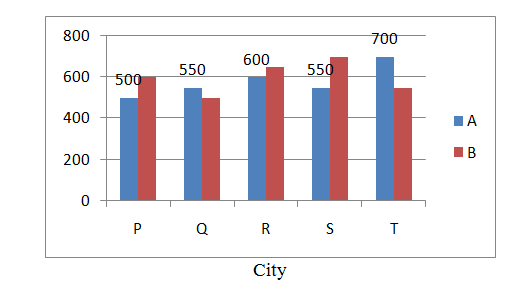New Students Offer - Use Code HELLO

# Data Interpretation Practice Set - 14

Directions (1 - 5): Study the following graph carefully and answer the questions that follow:
The graph given below represents the number of users of two broadband services
A and B across 5 cities P, Q, R, S and T.Q1. What is the total number of users of brand B across all give cities together?
a) 2700                                    b) 3000                                    c) 3100
d) 2900                                    e) 3200

Q2. The number of users of brand A in city T is what percent of the number of users of brand B in city Q?
a) 150                                       b) 110                           c) 140
d) 160                                       e) 120

Q3. What is the average number of users of brand A across all five cities together?
a) 560                                        b) 570                           c) 580
d) 590                                        e) 550

Q4. What is the difference between the total number of users of brand A and B together in city R and the ottal number of users of brand A and B together in city P?
a) 170                                        b) 140                            c) 130
d) 150                                        e) 160

Q5.  What is the respective ratio of the number users of brand A in city P to the number of users of brand B in city S?
a) 5 : 7                                   b) 4 : 7                         c) 2 : 5
d) 3 : 4                                    e) 5 : 6

Solution – 1 (Option B)
Total users of brand B across five cities = 600 + 500 + 650 + 700 + 550 = 3000

Solution – 2 (Option C)
Brand A users in city T = 700
Brand B users in city Q = 500
Required % = 700 / 500  × 100 = 140%

Solution – 3 (Option C)
Total users of Brand A across five cities = 500 + 550 + 600 + 550 + 700 = 2900

Average = 2900 / 5 = 580

Solution – 4 (Option D)
Brand A and B users in city R = 600 + 650 = 1250
Brand A and B users in city P = 500 + 600 = 1100
Required difference = 1250 1100 = 150

Solution – 5 (Option A )
Brand A users in city P = 500
Brand B users in city S = 700
Ratio = 500 / 700 = 5 / 7   = 5 : 7